In this notebook I decided to get more familiar with Julia basic functions, arrays, and operations by giving myself a small computational photography challenge! And on a suggestion, I played with the Interact.jl package too.

I’ve included the notebook I used for my image tiling manipulation, ending with playing with the Interact.jl library.

# My notebook

In this notebook I want to take an image, break it into regions, and tile it with the maximum color from that region. It is an experiment in computational photography!

In :
using Colors, Images, TestImages;


The one thing I find hard to get used to with Julia is that I don't need to explicitly call functions on module names, like in Python. Just a small detail, but I'll get used to it.

In :
img = testimage("lighthouse");


Here's some documentation about Arrays, which are what images are represented as: http://docs.julialang.org/en/stable/manual/arrays/

In :
# find the size of the image
println(size(img))

Out:
(512,768)

In :
summary(img)

Out:
"512×768 Array{RGB{N0f8},2}"
In :
# create a random dense array the same size as the image
# it gets initialized with random numbers, which makes a really cool pattern!
tiled_img = Array{RGB{N0f8}, 2}(size(img))

Out: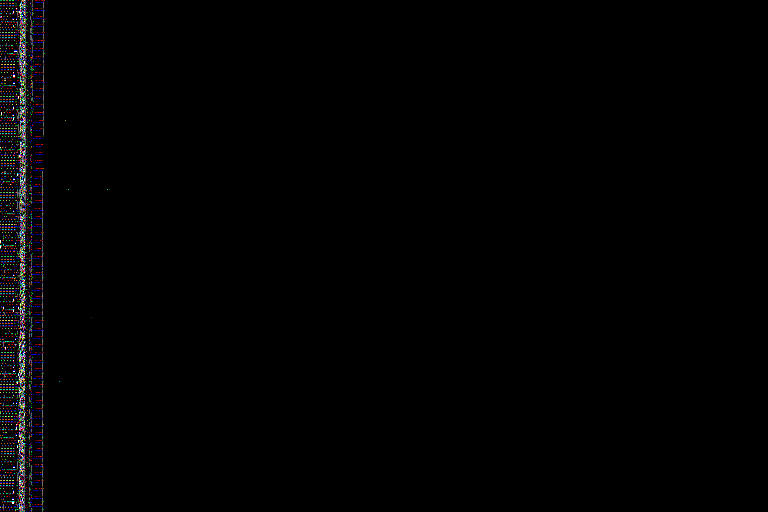Another way you can initialize an array like another:

In :
tiled_img = similar(img);


Like in IPython, you can add a "?" at the end of a function. In Julia, you put the question mark at the start of a function.

In :
?linspace

Out:
search:

# Iterating and Generating¶

There are many ways of generating a range of integers (that I will use to index into the image)

In :
linspace(1, size(img, 1), 8)

Out:
8-element LinSpace{Float64}:
1.0,74.0,147.0,220.0,293.0,366.0,439.0,512.0
In :
Int16.(linspace(1, size(img, 1), 8))

Out:
8-element Array{Int16,1}:
1
74
147
220
293
366
439
512
In :
Int16[i for i in linspace(1, size(img, 1), 8)]

Out:
8-element Array{Int16,1}:
1
74
147
220
293
366
439
512

You can generate x, y tile indices with an interator-like thing or a generator-like thing.

In :
# or to directly generate ints
1:8:size(img, 1)

Out:
1:8:505
In :
typeof(1:8:size(img, 1))

Out:
StepRange{Int64,Int64}

And the visualizations for strips of images is awesome!

In :
img[1:8:size(img, 1)]

Out:

If you use these generators to index in both directions, what it basically does is tile the image and downsample.

In :
stepsize = 16;
img[1:stepsize:size(img, 1), 1:stepsize:size(img, 2)]

Out: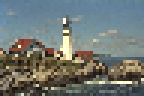In :
maximum(green.(img[1:10]))

Out:
0.518N0f8
In :
maximum(green.(img[1:16, 1:16]))

Out:
0.533N0f8

And now let's put it all together to take the max R, G, and B values in a block.

In :
for x in 1:stepsize:size(img, 1)
for y in 1:stepsize:size(img, 2)
x_end = min(x + stepsize, size(img, 1));
y_end = min(y + stepsize, size(img, 2));
# the @view takes a view into the image rather than making a copy
imgv = @view img[x:x_end, y:y_end]
tiled_img[x:x_end, y:y_end] = RGB{N0f8}(
maximum(red.(imgv)), maximum(green.(imgv)), maximum(blue.(imgv)));
end
end


And we can concatenate the images to put them size by side.

In :
[img tiled_img]

Out: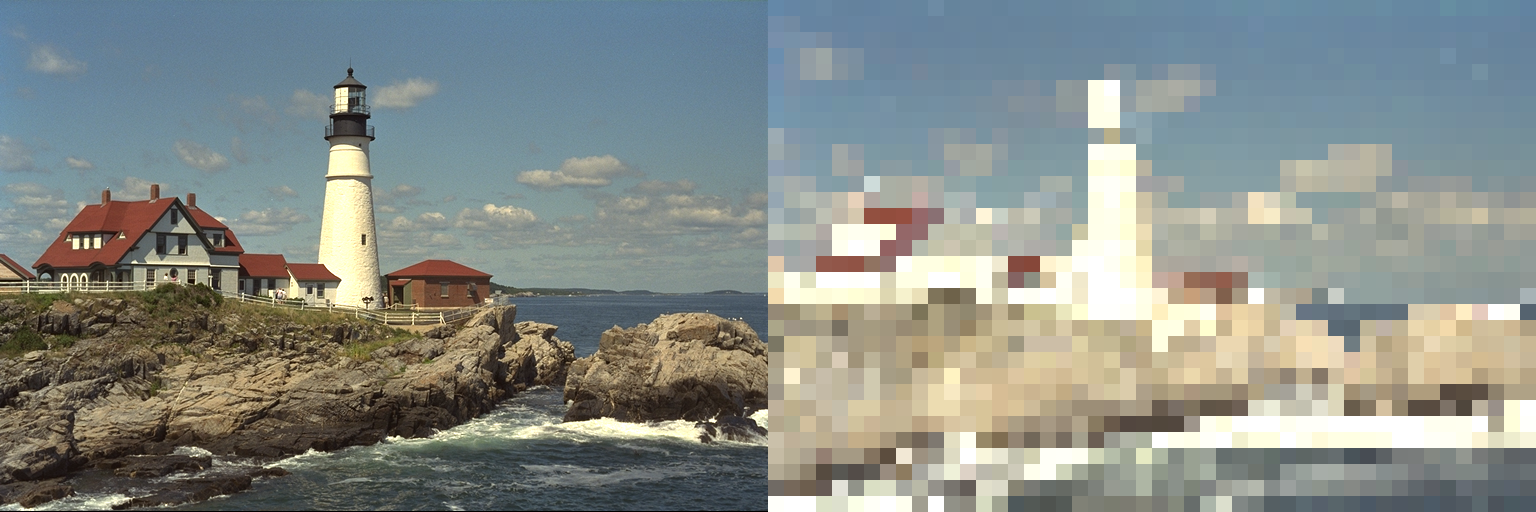I can even put all this into a function to call separately. Small notes about functions:

• Arguments can either be positional or keyword, but not both. The function below means I have to call stepsize= every time I want to pass stepsize.
• The last statement is the object returned by the function.
In :
function max_tiling(img; stepsize=16)
tiled_img = similar(img);
for x in 1:stepsize:size(img, 1)
for y in 1:stepsize:size(img, 2)
x_end = min(x + stepsize, size(img, 1));
y_end = min(y + stepsize, size(img, 2));
imgv = @view img[x:x_end, y:y_end];
tiled_img[x:x_end, y:y_end] = RGB{N0f8}(
maximum(red.(imgv)), maximum(green.(imgv)), maximum(blue.(imgv)));
end
end
tiled_img
end

Out:
max_tiling (generic function with 1 method)
In :
[img max_tiling(img, stepsize=16)]

Out: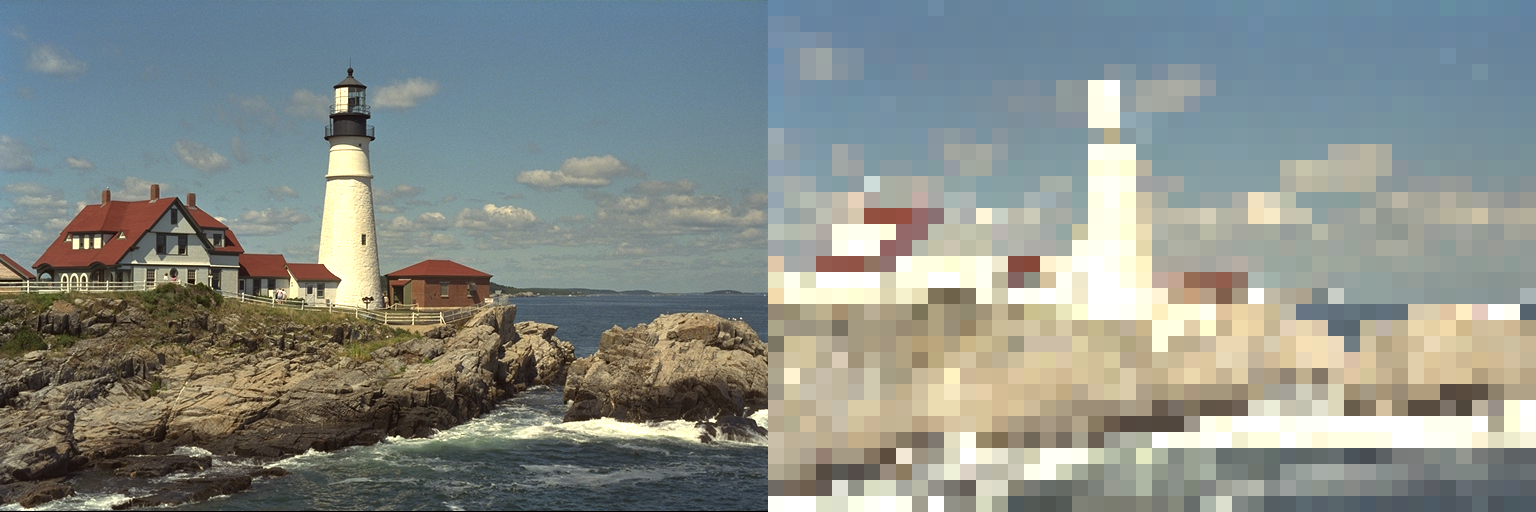In :
[img max_tiling(img, stepsize=8)]

Out:I am told that the interact.jl package (https://github.com/JuliaGizmos/Interact.jl) can let me make a slider to change the stepsize!

In :
for x in 1:stepsize:size(img, 1)
for y in 1:stepsize:size(img, 2)
x_end = min(x + stepsize, size(img, 1));
y_end = min(y + stepsize, size(img, 2));
imgv = @view img[x:x_end, y:y_end]
tiled_img[x:x_end, y:y_end] = RGB{N0f8}(maximum(red.(imgv)), maximum(green.(imgv)), maximum(blue.(imgv)));
end
end

In :
# Pkg.add("Interact")

In :
using Interact;

Out:

The widget lets me manipulate the step size and re-generate images directly in the notebook! Highly recommend this package =)

In :
@manipulate for s=[2^x for x in 0:6]
[img max_tiling(img, stepsize=s)]
end

Out:

The widget in the last output of the notebook did not render properly, so I’m including a screenshot here: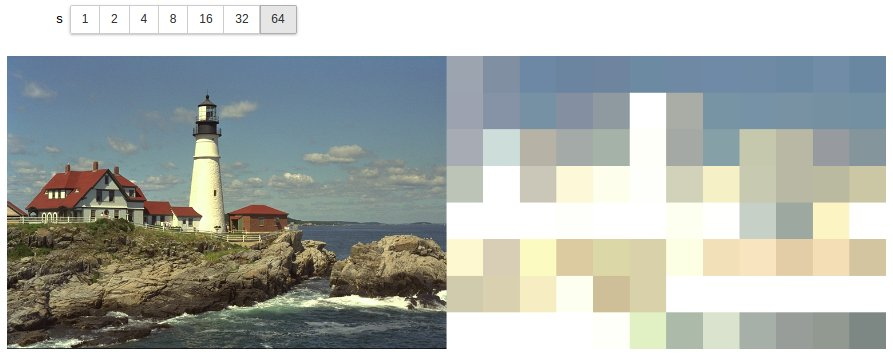# Random memory initialization

In line 4 (In:), an uninitialized array takes random memory and random values. I don’t fully understand where this memory comes from, but I’m pretty sure it has to do with most-recently accessed memory. When I was running on my local machine, line 2 (In:) loaded the original image of the lighthouse we saw in my first post. So when line 4 (creating a random uninitialized array) was executed, I got this pretty lighthouse artifact! You can see it is different image than came up in the second run of the notebook rendered above!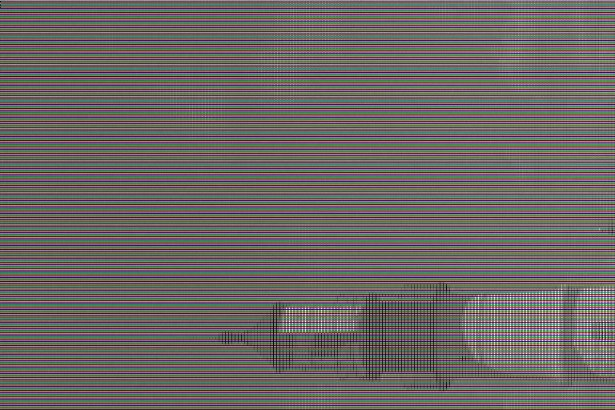# Jupyter notebook rendering aside

As another aside, in rendering the Jupyter notebook, the Interact library created widgets that generated custom Javascript during the nbconvert phase. I just removed that entire script section from the generated HTML, which is why I had to include a screenshot of the final widget at the end. I’m sorry for that ugliness - Jupyter and nbconvert are fantastic projects, they just can’t possibly cover all use cases!